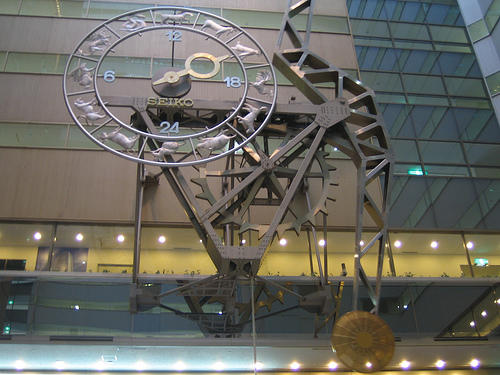## Time

(photo by  Colin McMillen used under terms of Creative Commons license.)Section 1

You  will hear  10 situations.  Respond  to  each  in  Japanese. After  you  respond,  the  correct  answer  will be  heard  on  the tape.  On  a  separate  piece  of  paper,  keep  track  of  which items  you  miss  and  which  you  answer  correctly.

Section 2

You  will hear  16  statements  in  Japanese.  Select  the correct  English  equivalent  for  each  sentence  from  the  three choices  provided,  and  write  A,  B,  or  C  on  a  separate  piece  of paper.  After  you  finish  this  section,  check  your  answers  with the  key.

1.

A .  It's  3:10.

B .  It's  3:20.

C .  It's  3:50.

2.

A.  It's  1:30  now.

B .  It's  7:30  now.

C .  It's  8:30  now.

3.

A .  It's  15  after  6 .

B .  It's  15  to  7 .

C .  It's  15  after  7 .

4.

A .  It's  2:20.

B .  It's  11: 30.

C .  It's  12:40.

5.

A .  It's  5:10  now.

B .  It's  5:20  now.

C .  It's  5:30  now.

6.

A .  It's  6:20,

B .  It's  9:10,

C .  It's  9:20.

7.

A .  It's  20  to  4 .

B .  It's  10  to  4 .

C.  It's  10  after  4 .

8.

A .  It's  1:15.

B .  It's  11:15.

C .  It's  11:45.

9.

A .  It's  1:25.

B .  It's  8:15.

C .  It's  8:55.

10.

A .  We  leave  at  6: 18.

B .  We  leave  at  6:28.

C .  We  leave  at  9:08.

11.

We  arrive  at  5: 16.

B .  We  arrive  at  6:29.

C .  We  arrive  at  9:26.

12.

A .  We  leave  at  15  to  7 .

B .  We  leave  at  5  to  7 .

C .  We  leave  at  5  after  7 .

13.

A.  We  arrive  around  two  o'clock  today.

B .  We  arrive  around  ten  o'clock  today.

C .  We  arrive  around  twelve  o'clock  today.

14.

A .  We  arrive  in  about  another  ten  minutes.

B .  We  leave  in  about  another  ten  minutes.

C .  We  leave  i n  about  another  twenty  minutes.

15.

A .  We  arrive  around  ten  o'clock  tomorrow.

B .  We  arrive  around  eleven  o'clock  tomorrow.

C .  We  arrive  around  twelve  o'clock  tomorrow.

16.

A .  We  arrive  in  about  another  five  minutes.

B .  We  arrive  in  about  another  fifteen  minutes.

C .  We  leave  in  about  another  fifteen  minutes.

Key  to  Section 2:

 1.  A 5.  A 9.  C 13.  A 2.  C 6.  C 10.  A 14.  B 3.  B 7.  B 11.  C 15.  A 4.  C 8.  B 12.  B 16.  C